# GMAT Math : DSQ: Calculating the length of an arc

## Example Questions

### Example Question #1 : Dsq: Calculating The Length Of An Arc

How far has the tip of the hour hand of a clock traveled since noon?

1) It is now 5:00 PM.

2) The hour hand is half the length of the minute hand.

Statement 2 ALONE is sufficient to answer the question, but Statement 1 ALONE is not sufficient.

BOTH statements TOGETHER are NOT sufficient to answer the question.

EITHER Statement 1 ALONE or Statement 2 ALONE is sufficient to answer the question.

Statement 1 ALONE is sufficient to answer the question, but Statement 2 ALONE is not sufficient.

BOTH statements TOGETHER are sufficient to answer the question, but NEITHER statement ALONE is sufficient to answer the question.

BOTH statements TOGETHER are NOT sufficient to answer the question.

Explanation:

The time alone is insufficient without the length of the hand. The second statement does not give us that information, only the relationship between the lengths of the two hands, which is useless without the length of the minute hand.

The answer is that both statements together are insufficient to answer the question.

### Example Question #2 : Dsq: Calculating The Length Of An Arc

Arc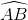is located on circle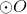;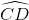is located on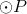. Which arc, if either, has greater degree measure?

Statement 1:andhave the same length.

Statement 2: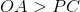BOTH statements TOGETHER are sufficient to answer the question, but NEITHER statement ALONE is sufficient to answer the question.

BOTH statements TOGETHER are insufficient to answer the question.

EITHER statement ALONE is sufficient to answer the question.

Statement 2 ALONE is sufficient to answer the question, but Statement 1 ALONE is NOT sufficient to answer the question.

Statement 1 ALONE is sufficient to answer the question, but Statement 2 ALONE is NOT sufficient to answer the question.

BOTH statements TOGETHER are sufficient to answer the question, but NEITHER statement ALONE is sufficient to answer the question.

Explanation:

The arc with the greater degree measure is the one which is the greater part of its circle.

If both arcs have the same length, then the one that is the greater part of its circle must be the one on the smaller circle; Statement 1 alone tells us both have the same length, but not which circle is smaller.

If, thenhas the greater radius and, sqbsequently, the greater circumference; it is the larger circle. But we know nothing about the measures of the arcs, so Statement 2 alone is insufficient.

If we know both statements, however, we know that, since the arcs have the same length, andis the larger circle - with greater circumference -must be take up the lesser portion of its circle, and have the lesser degree measure of the two.

### Example Question #3 : Dsq: Calculating The Length Of An Arc

Arcis located on circle;is located onWhich arc, if either, is longer?

Statement 1: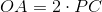Statement 2: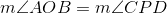BOTH statements TOGETHER are sufficient to answer the question, but NEITHER statement ALONE is sufficient to answer the question.

Statement 1 ALONE is sufficient to answer the question, but Statement 2 ALONE is NOT sufficient to answer the question.

BOTH statements TOGETHER are insufficient to answer the question.

EITHER statement ALONE is sufficient to answer the question.

Statement 2 ALONE is sufficient to answer the question, but Statement 1 ALONE is NOT sufficient to answer the question.

BOTH statements TOGETHER are sufficient to answer the question, but NEITHER statement ALONE is sufficient to answer the question.

Explanation: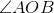and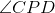are the central angles that interceptand, respectively. The measure of an arc is equal to that of its central angle, so, is we are given Statement 2, that, we know that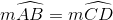. The arcs are the same portion of their respective circles. The larger ofanddetermines which arc is longer; this is given in Statement 1, since, if, thenhas the greater radius and circumference.

Both statements together are sufficient to show thatis the longer of the two, but neither alone is suffcient. From Statement 1, the relative sizes of the circles are known, but not the degree measures of the arcs; it is possible for an arc on a larger circle to have length less than, equal to, or greater than the arc on the smaller circle. From Statement 2 alone, the degree measures of the arcs can be proved equal, but not thier lengths.

### Example Question #4 : Dsq: Calculating The Length Of An Arc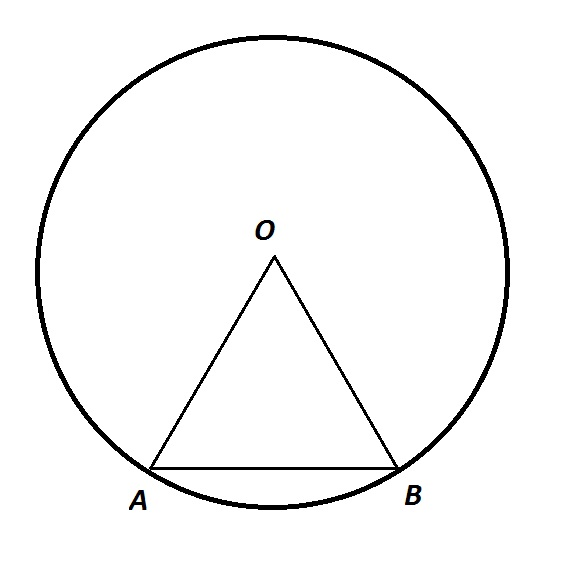Note: Figure NOT drawn to scale.

In the above figure,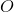is the center of the circle. Give the length of arc.

Statement 1: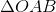is an equilateral triangle.

Statement 2:has area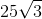.

BOTH statements TOGETHER are sufficient to answer the question, but NEITHER statement ALONE is sufficient to answer the question.

Statement 2 ALONE is sufficient to answer the question, but Statement 1 ALONE is NOT sufficient to answer the question.

BOTH statements TOGETHER are insufficient to answer the question.

EITHER statement ALONE is sufficient to answer the question.

Statement 1 ALONE is sufficient to answer the question, but Statement 2 ALONE is NOT sufficient to answer the question.

BOTH statements TOGETHER are sufficient to answer the question, but NEITHER statement ALONE is sufficient to answer the question.

Explanation:

From Statement 1 alone,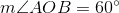, socan be determined to be aarc. But no method is given to find the length of the arc.

From Statement 2 alone, neither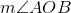nor radius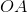can be determined, as the area of a triangle alone cannot be used to determine any angle or side.

From the two statements together,, and the common sidelength of the equilateral triangle can be determined from the formula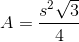This sidelengthis the radius of the circle. Onceis calculated, the circumference can be calculated, and the arc length will be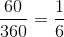of this.

### Example Question #5 : Dsq: Calculating The Length Of An Arc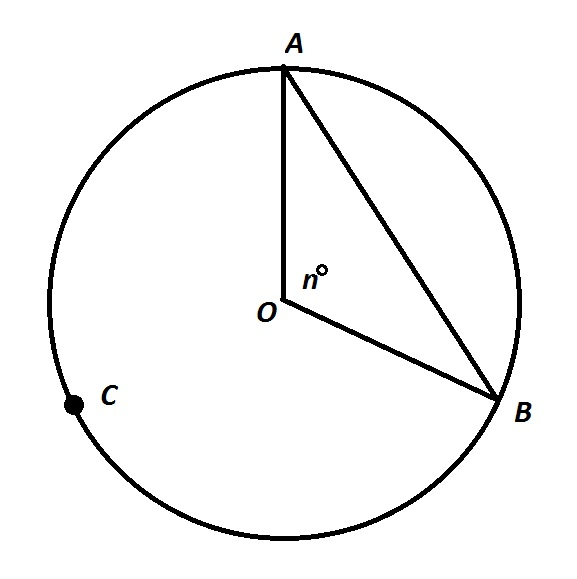Note: Figure NOT drawn to scale.

In the above figure,is the center of the circle. Give the length of arc.

Statement 1: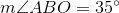Statement 2: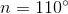BOTH statements TOGETHER are sufficient to answer the question, but NEITHER statement ALONE is sufficient to answer the question.

Statement 2 ALONE is sufficient to answer the question, but Statement 1 ALONE is NOT sufficient to answer the question.

Statement 1 ALONE is sufficient to answer the question, but Statement 2 ALONE is NOT sufficient to answer the question.

BOTH statements TOGETHER are insufficient to answer the question.

EITHER statement ALONE is sufficient to answer the question.

BOTH statements TOGETHER are insufficient to answer the question.

Explanation:

If either or both Statement 1 and Statement 2 are known, then then only thing aboutthat can be determined is that it is an arc of measure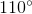. Without knowing any of the linear measures of the circle, such as the radius or the circumference, it is impossible to determine the length of.

### Example Question #6 : Dsq: Calculating The Length Of An ArcNote: Figure NOT drawn to scale.

In the above figure,is the center of the circle. Give the length of arc.

Statement 1: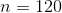Statement 2: Major arc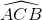has length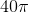.

BOTH statements TOGETHER are sufficient to answer the question, but NEITHER statement ALONE is sufficient to answer the question.

Statement 2 ALONE is sufficient to answer the question, but Statement 1 ALONE is NOT sufficient to answer the question.

Statement 1 ALONE is sufficient to answer the question, but Statement 2 ALONE is NOT sufficient to answer the question.

EITHER statement ALONE is sufficient to answer the question.

BOTH statements TOGETHER are insufficient to answer the question.

BOTH statements TOGETHER are sufficient to answer the question, but NEITHER statement ALONE is sufficient to answer the question.

Explanation:

Statement 1 only establishes thatis one-third of the circle. Without other information such as the radius, the circumference, or the length of an arc, it is impossible to determine the length of the chord. Statement 2 alone is also insufficient to give the length of the chord, for similar reasons.

The two statements together, however, establish thatis the length of the major arc of a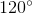central angle, and therefore, two-thirds the circumference. The circumference can therefore be calculated to be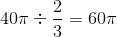, and minor arcis one third of this, or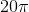.

### Example Question #7 : Dsq: Calculating The Length Of An Arc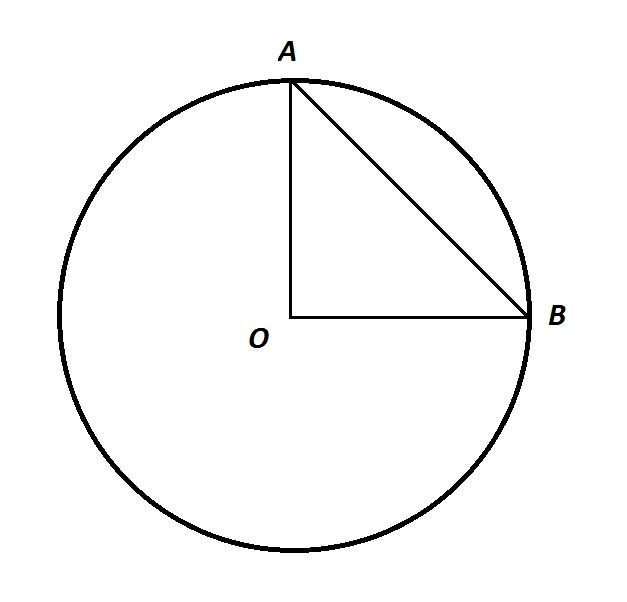Note: Figure NOT drawn to scale.

In the above figure,is the center of the circle, and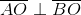. Give the length of arc.

Statement 1: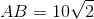.

Statement 2: The area of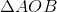is.

BOTH statements TOGETHER are sufficient to answer the question, but NEITHER statement ALONE is sufficient to answer the question.

Statement 1 ALONE is sufficient to answer the question, but Statement 2 ALONE is NOT sufficient to answer the question.

BOTH statements TOGETHER are insufficient to answer the question.

EITHER statement ALONE is sufficient to answer the question.

Statement 2 ALONE is sufficient to answer the question, but Statement 1 ALONE is NOT sufficient to answer the question.

EITHER statement ALONE is sufficient to answer the question.

Explanation:, makinga right triangle. Since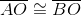, both segments being radii,is also a 45-45-90 triangle.

From Statement 1 alone, it can be determined by way of the 45-45-90 Theorem that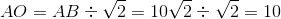.

From Statement 2 alone, since the area of a right triangle is half the product of its legs,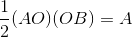Since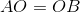and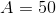,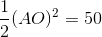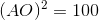From either statement alone, the radius of the circle can be calculated. From there, the circumference can be calculated, and the length of the arc can be found by multiplying the circumference by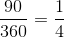Tired of practice problems?

Try live online GMAT prep today.